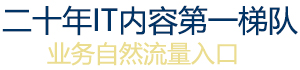﻿ Heap buffer overflowkaldi数据准备

kaldi开发技术服务

Java 安卓移动开发
VC++
C#软件

Q Q：396806883

# 从NSInteger到NSNumber的隐含转换被禁止

## 问

I've written this piece of code:我写了这段代码：

```    for (int i = 1; i < 1000; i++) {
for (int j = 1; j < 1000; j++) {
int k = i * j;                //This is the line were the error messages appears
NSString *number = [NSString stringWithFormat:@"%d", k];
NSNumber *length = number.length;
NSNumber *half = (length / 2);  ```

What I want it to do, is to take the int k, convert it into a NSString named number, store the length of the string number in the NSNumber length, and half of that value in the NSNumber half.

However, when trying to convert the int k to the NSString number I get the error message:

"Implicit conversion of 'NSInteger' (aka 'unsigned int') to 'NSNumber *' is disallowed with arc"从NSInteger到NSNumber的隐含转换被禁止

Could someone help me figure out why?

## 答

number.length returns integer not NSNumber.number.length返回整数而不是NSNumber。

Correct it to :纠正它：

```NSInteger length = number.length;
NSInteger half = (length / 2);  ```

If you really want to use NSNumber do like this: (Here I am boxing the integer to NSNumber object)如果你真的想使用NSNumber这样做:(这里我将整数装箱到NSNumber对象）

```NSNumber *length = @(number.length); //same as [NSNumber numberWithInt:number.length]
NSNumber *half = @(number.length / 2);```